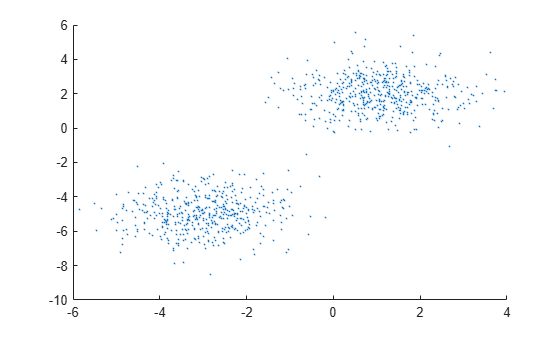Documentation

# random

Random variate from Gaussian mixture distribution

## Syntax

``Y = random(gm)``
``Y = random(gm,n)``
``[Y,compIdx] = random(___)``

## Description

example

````Y = random(gm)` generates a 1-by-m random variate from the m-dimensional Gaussian mixture distribution `gm`.```

example

````Y = random(gm,n)` returns `n` random variates. Each row of `Y` is a random variate generated from the m-dimensional Gaussian mixture distribution `gm`.```

example

````[Y,compIdx] = random(___)` also returns an `n`-by-1 index vector `compIdx` for any of the input arguments in previous syntaxes. `compIdx(i)` indicates the mixture component used to generate the `i`th random variate `Y(i,:)`.```

## Examples

collapse all

Create a `gmdistribution` object and generate random variates.

Define the distribution parameters (means and covariances) of a two-component bivariate Gaussian mixture distribution.

```mu = [1 2;-3 -5]; sigma = [1 1]; % shared diagonal covariance matrix```

Create a `gmdistribution` object by using the `gmdistribution` function. By default, the function creates an equal proportion mixture.

`gm = gmdistribution(mu,sigma)`
```gm = Gaussian mixture distribution with 2 components in 2 dimensions Component 1: Mixing proportion: 0.500000 Mean: 1 2 Component 2: Mixing proportion: 0.500000 Mean: -3 -5 ```

Generate 1000 random variates.

```rng('default'); % For reproducibility [Y,compIdx] = random(gm,1000);```

`compIdx(i)` indicates the mixture component used to generate the `i`th random variate `Y(i,:)`. Count the number of random variates generated by `Component` `1`.

`numIdx1 = sum(compIdx == 1)`
```numIdx1 = 512 ```

`random` generates about half of the random variates using `Component` `1` because `gm` has equal mixing proportions.

Plot the generated random variates by using `scatter`.

`scatter(Y(:,1),Y(:,2),10,'.') % Scatter plot with points of size 10`Reset the random number generator to generate the same random variate.

Define the distribution parameters (means, covariances, and mixing proportions) of two bivariate Gaussian mixture components.

```p = [0.4 0.6]; % Mixing proportions mu = [1 2;-3 -5]; % Means sigma = cat(3,[2 .5],[1 1]) % Covariances 1-by-2-by-2 array```
```sigma = sigma(:,:,1) = 2.0000 0.5000 sigma(:,:,2) = 1 1 ```

The `cat` function concatenates the covariances along the third array dimension. The defined covariance matrices are diagonal matrices. `sigma(1,:,i)` contains the diagonal elements of the covariance matrix of component `i`.

Create a `gmdistribution` object by using the `gmdistribution` function.

`gm = gmdistribution(mu,sigma);`

Save the current state of the random number generator, and then generate a random variate using `gm`.

```s = rng; r = random(gm)```
```r = 1×2 -1.1661 -7.2588 ```

Restore the state of the random number generator to `s`, and then generate a random variate using `gm`. The values are the same as before.

```rng(s); r1 = random(gm)```
```r1 = 1×2 -1.1661 -7.2588 ```

## Input Arguments

collapse all

Gaussian mixture distribution, also called Gaussian mixture model (GMM), specified as a `gmdistribution` object.

You can create a `gmdistribution` object using `gmdistribution` or `fitgmdist`. Use the `gmdistribution` function to create a `gmdistribution` object by specifying the distribution parameters. Use the `fitgmdist` function to fit a `gmdistribution` model to data given a fixed number of components.

Number of random variates to generate, specified as a positive integer.

Data Types: `single` | `double`

## Output Arguments

collapse all

Random variate, returned as a 1-by-m numeric vector or an `n`-by-m numeric matrix. Each row of `Y` is a random variate generated from the m-dimensional Gaussian mixture distribution `gm`.

Component index, returned as a positive integer or an `n`-by-1 index vector, where `compIdx(i)` indicates the mixture component used to generate the `i`th random variate `Y(i,:)`.